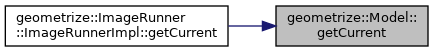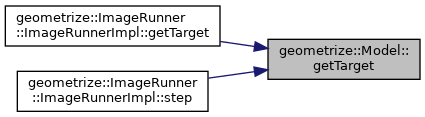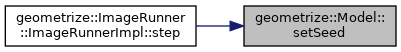Geometrize  1.0 C++ library for geometrizing images into geometric primitives
geometrize::Model Class Reference

The Model class is the model for the core optimization/fitting algorithm. More...

`#include <model.h>`

class  ModelImpl

## Public Member Functions

Model (const geometrize::Bitmap &target)
Model Creates a model that will aim to replicate the target bitmap with shapes. More...

Model (const geometrize::Bitmap &target, const geometrize::Bitmap &initial)
Model Creates a model that will optimize for the given target bitmap, starting from the given initial bitmap. The target bitmap and initial bitmap must be the same size (width and height). More...

~Model ()

Modeloperator= (const Model &)=delete

Model (const Model &)=delete

void reset (geometrize::rgba backgroundColor)
reset Resets the model back to the state it was in when it was created. More...

std::int32_t getWidth () const
getWidth Gets the width of the target bitmap. More...

std::int32_t getHeight () const
getHeight Gets the height of the target bitmap. More...

std::vector
< geometrize::ShapeResult
step (const std::function< std::shared_ptr< geometrize::Shape >(void)> &shapeCreator, std::uint8_t alpha, std::uint32_t shapeCount, std::uint32_t maxShapeMutations, std::uint32_t maxThreads, const geometrize::core::EnergyFunction &energyFunction=nullptr)
step Steps the primitive optimization/fitting algorithm. More...

geometrize::ShapeResult drawShape (std::shared_ptr< geometrize::Shape > shape, geometrize::rgba color)
drawShape Draws a shape on the model. Typically used when to manually add a shape to the image (e.g. when setting an initial background). NOTE this unconditionally draws the shape, even if it increases the difference between the source and target image. More...

geometrize::BitmapgetCurrent ()
getCurrent Gets the current bitmap. More...

geometrize::BitmapgetTarget ()
getTarget Gets the target bitmap. More...

const geometrize::BitmapgetCurrent () const
getCurrent Gets the current bitmap, const-edition. More...

const geometrize::BitmapgetTarget () const
getTarget Gets the target bitmap, const-edition. More...

void setSeed (std::uint32_t seed)
setSeed Sets the seed that the random number generators of this model use. Note that the model also uses an internal seed offset which is incremented when the model is stepped. More...

## Private Attributes

std::unique_ptr< Model::ModelImpld

## Detailed Description

The Model class is the model for the core optimization/fitting algorithm.

## Constructor & Destructor Documentation

 geometrize::Model::Model ( const geometrize::Bitmap & target )

Model Creates a model that will aim to replicate the target bitmap with shapes.

Parameters
 target The target bitmap to replicate with shapes.
201  : d{std::unique_ptr<Model::ModelImpl>(new Model::ModelImpl(target))}
202 {}
std::unique_ptr< Model::ModelImpl > d
Definition: model.h:120
 geometrize::Model::Model ( const geometrize::Bitmap & target, const geometrize::Bitmap & initial )

Model Creates a model that will optimize for the given target bitmap, starting from the given initial bitmap. The target bitmap and initial bitmap must be the same size (width and height).

Parameters
 target The target bitmap to replicate with shapes. initial The starting bitmap.
204  : d{std::unique_ptr<Model::ModelImpl>(new Model::ModelImpl(target, initial))}
205 {}
std::unique_ptr< Model::ModelImpl > d
Definition: model.h:120
 geometrize::Model::~Model ( )
208 {}
 geometrize::Model::Model ( const Model & )
delete

## Member Function Documentation

 geometrize::ShapeResult geometrize::Model::drawShape ( std::shared_ptr< geometrize::Shape > shape, geometrize::rgba color )

drawShape Draws a shape on the model. Typically used when to manually add a shape to the image (e.g. when setting an initial background). NOTE this unconditionally draws the shape, even if it increases the difference between the source and target image.

Parameters
 shape The shape to draw. color The color (including alpha) of the shape.
Returns
Data about the shape drawn on the model.
237 {
238  return d->drawShape(shape, color);
239 }
std::unique_ptr< Model::ModelImpl > d
Definition: model.h:120
 geometrize::Bitmap & geometrize::Model::getCurrent ( )

getCurrent Gets the current bitmap.

Returns
The current bitmap.
247 {
248  return d->getCurrent();
249 }
std::unique_ptr< Model::ModelImpl > d
Definition: model.h:120

Here is the caller graph for this function:const geometrize::Bitmap & geometrize::Model::getCurrent ( ) const

getCurrent Gets the current bitmap, const-edition.

Returns
The current bitmap.
257 {
258  return d->getCurrent();
259 }
std::unique_ptr< Model::ModelImpl > d
Definition: model.h:120
 std::int32_t geometrize::Model::getHeight ( ) const

getHeight Gets the height of the target bitmap.

Returns
The height of the target bitmap.
221 {
222  return d->getHeight();
223 }
std::unique_ptr< Model::ModelImpl > d
Definition: model.h:120
 geometrize::Bitmap & geometrize::Model::getTarget ( )

getTarget Gets the target bitmap.

Returns
The target bitmap.
242 {
243  return d->getTarget();
244 }
std::unique_ptr< Model::ModelImpl > d
Definition: model.h:120

Here is the caller graph for this function:const geometrize::Bitmap & geometrize::Model::getTarget ( ) const

getTarget Gets the target bitmap, const-edition.

Returns
The target bitmap.
252 {
253  return d->getTarget();
254 }
std::unique_ptr< Model::ModelImpl > d
Definition: model.h:120
 std::int32_t geometrize::Model::getWidth ( ) const

getWidth Gets the width of the target bitmap.

Returns
The width of the target bitmap.
216 {
217  return d->getWidth();
218 }
std::unique_ptr< Model::ModelImpl > d
Definition: model.h:120
 Model& geometrize::Model::operator= ( const Model & )
delete
 void geometrize::Model::reset ( geometrize::rgba backgroundColor )

reset Resets the model back to the state it was in when it was created.

Parameters
 backgroundColor The starting background color to use.
211 {
212  d->reset(backgroundColor);
213 }
std::unique_ptr< Model::ModelImpl > d
Definition: model.h:120
 void geometrize::Model::setSeed ( std::uint32_t seed )

setSeed Sets the seed that the random number generators of this model use. Note that the model also uses an internal seed offset which is incremented when the model is stepped.

Parameters
 seed The random number generator seed.
262 {
263  d->setSeed(seed);
264 }
std::unique_ptr< Model::ModelImpl > d
Definition: model.h:120

Here is the caller graph for this function:std::vector< geometrize::ShapeResult > geometrize::Model::step ( const std::function< std::shared_ptr< geometrize::Shape >(void)> & shapeCreator, std::uint8_t alpha, std::uint32_t shapeCount, std::uint32_t maxShapeMutations, std::uint32_t maxThreads, const geometrize::core::EnergyFunction & energyFunction = `nullptr` )

step Steps the primitive optimization/fitting algorithm.

Parameters
 shapeCreator A function that will produce the shapes. alpha The alpha of the shape. shapeCount The number of random shapes to generate (only 1 is chosen in the end). maxShapeMutations The maximum number of times to mutate each random shape. maxThreads The maximum number of threads to use during this step. energyFunction An optional function to calculate the energy (if unspecified a default implementation is used).
Returns
A vector containing data about the shapes added to the model in this step. This may be empty if no shape that improved the image could be found.
232 {
233  return d->step(shapeCreator, alpha, shapeCount, maxShapeMutations, maxThreads, energyFunction);
234 }
std::unique_ptr< Model::ModelImpl > d
Definition: model.h:120

Here is the caller graph for this function:## Member Data Documentation

 std::unique_ptr geometrize::Model::d
private

The documentation for this class was generated from the following files:
• /home/travis/build/Tw1ddle/geometrize-lib-docs/geometrize/geometrize/geometrize/model.h
• /home/travis/build/Tw1ddle/geometrize-lib-docs/geometrize/geometrize/geometrize/model.cpp